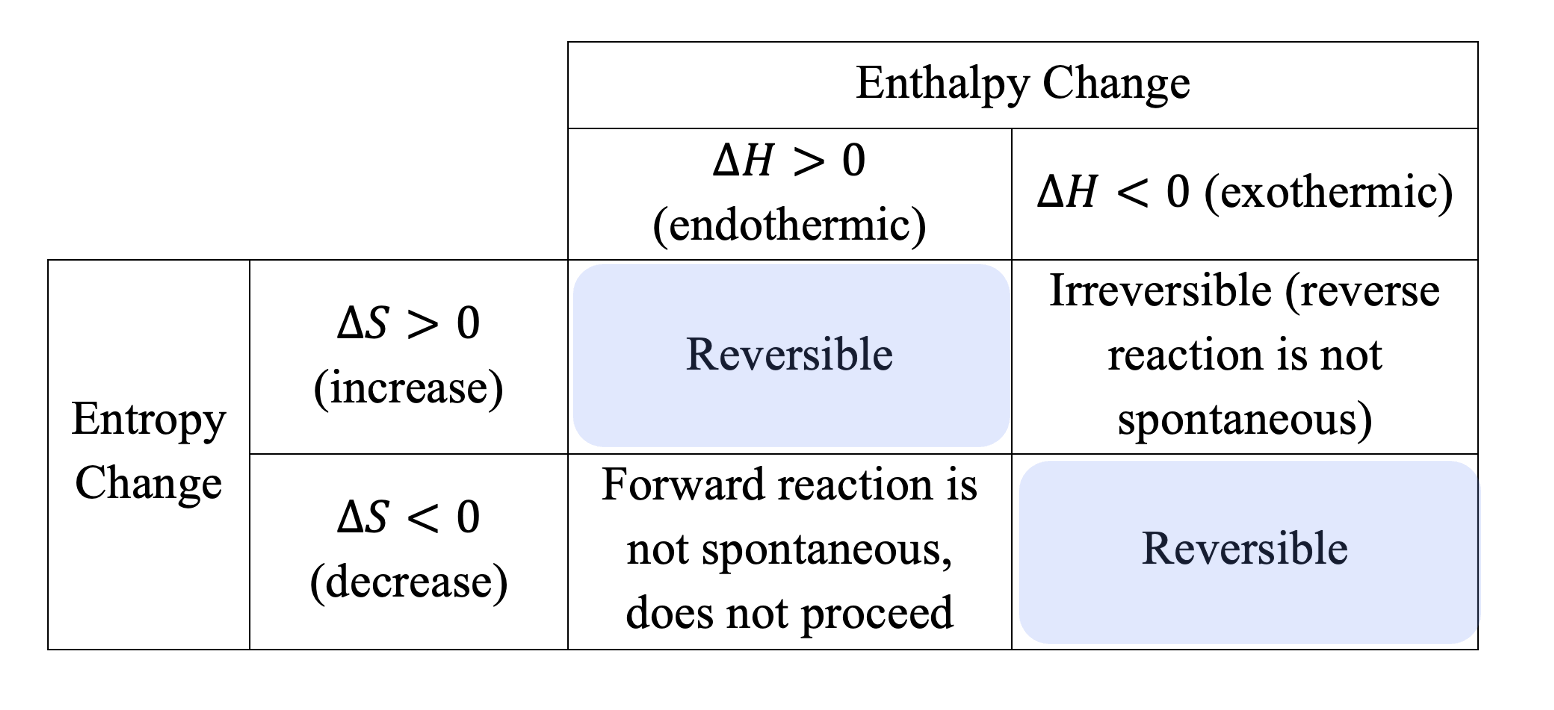# Entropy and Gibbs Free Energy

This is part of Year 11 HSC Chemistry course under the topic of Entropy and Gibbs Free Energy

### HSC Chemistry Syllabus

• Analyse the differences between entropy and enthalpy

• Use modelling to illustrate entropy changes in reactions

• Predict entropy changes from balanced chemical reactions to classify as increasing or decreasing entropy

• Explain reaction spontaneity using terminology, including

– Gibbs Free Energy

– Entropy

### Entropy and Gibbs Free Energy

This video will discuss the concept of Entropy and also explain how the combination of entropy and enthalpy values can be utilised along with the Gibbs Free Energy equation to predict the spontaneity of a reaction.

### What is Entropy?

Entropy is a fundamental concept in chemistry which is often described as a measure of the chaos or "disordered-ness" of a system. In technical terms, we measure entropy in joules per kelvin per moles. But what does this mean in a practical sense?

Chemical systems can exhibit changes in entropy. When entropy increases, the system becomes more disordered. Conversely, a decrease in entropy signifies a more ordered state.

Examples:

Consider the melting of a solid into a liquid. This process increases entropy, indicating a move towards greater disorder.

The decomposition of calcium carbonate into solid calcium oxide and carbon dioxide gas increases entropy as a solid turns into a gas.### Entropy and Reaction Favorability

In the realm of chemistry, reactions often 'prefer' to move towards greater disorder, akin to how it's easier to make a room messy than to tidy it up. We describe this tendency using the term "favourability"

• Positive \Delta S: Indicates a reaction moving towards disordered, deemed to be favourable.
• Negative \Delta S: Suggests a move towards order, considered to be less favourable or unfavourable.

### Gibbs Free Energy: Combining Entropy and Enthalpy

Gibbs Free energy (G) is a critical concept that combines enthalpy (H) and entropy (S) to predict reaction spontaneity. Its equation is:

$$\Delta G = \Delta H – T \Delta S$$

Here \Delta G represents Gibbs Free Energy change, \Delta H is enthalpy change, T is temperature in Kelvin, and \Delta S is entropy change.Previous Section: Hess's Law